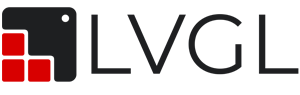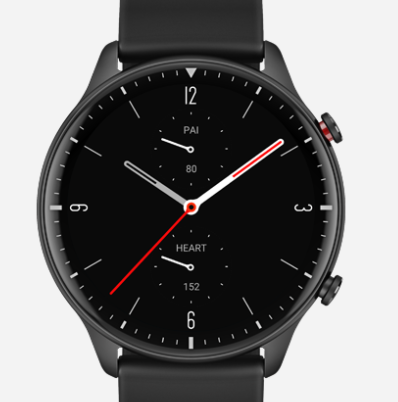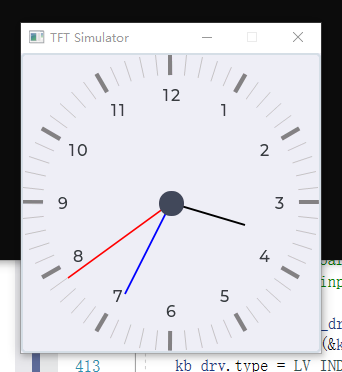# A widget for the dial of a watch

Stacking the meter into the dial (I need three hands of different lengths and two scales) seems a bit cumbersome, so it’s particularly desirable that LVGL will consider adding a dial widget in the near future

Could you send an image to better understand what you imagined?

Looks like the translator is not very accurate, I mean, I need to draw a watch dial for smart watches, similar to figure 1, but there is no corresponding widget can achieve directly, so I refer to guage.c and modify, effect is probably as shown in figure 2, if there is a watch dial widget (like guage widget) can call a function directly, will be very convenient. In summary, it is hoped that the future effect of Figure 1 can be achieved with a widget.(This paragraph is still a translation. I don’t know if it is clearly described. Thank you very much for your patience.)Figure 1Figure 2

I see thank you.
Noted!

i want the same widget too, suach as TouchGFX’s CLOCK widget.

how did you set the needle to different lenth,i have try to use img to set the needle,but only could be seted the same pictrue

I copied guage.c and modified it, such as the lv_gauge_draw_needle() function, then added it as a widget of my own, similar modifications can also make different Pointers to different images. The modification looks something like this:

static void lv_dial_draw_needle(lv_obj_t* dial, const lv_area_t* clip_area)
{
lv_dial_ext_t* ext = lv_obj_get_ext_attr(dial);

``````lv_style_int_t pad = lv_obj_get_style_pad_inner(dial, LV_DIAL_PART_NEEDLE);

lv_coord_t r = (lv_obj_get_width(dial) - left - right) / 2 - pad;
lv_coord_t r_needle;     //三根指针长度不一，各自分开储存   //----- 增加 - By XXX 20201116
for (uint8_t needle_id = 0; needle_id < 3; needle_id++) //将 needle_pad 代入计算   //----- 增加 - By XXX 20201116
{
r_needle[needle_id] = (lv_obj_get_width(dial) - left - right) / 2 - needle_pad[needle_id];
}
lv_coord_t x_ofs = dial->coords.x1 + r + left + pad;
lv_coord_t y_ofs = dial->coords.y1 + r + top + pad;
uint16_t angle = lv_linemeter_get_scale_angle(dial);
int16_t angle_ofs = 90 + (360 - angle) / 2 + lv_dial_get_angle_offset(dial);
int16_t min = lv_dial_get_min_value(dial);
int16_t max = lv_dial_get_max_value(dial);
lv_point_t p_mid;
lv_point_t p_end;
uint8_t i;

lv_draw_line_dsc_t line_dsc;
lv_draw_img_dsc_t img_dsc;
if (ext->needle_img == NULL) {
lv_draw_line_dsc_init(&line_dsc);
lv_obj_init_draw_line_dsc(dial, LV_DIAL_PART_NEEDLE, &line_dsc);
}
else {
lv_draw_img_dsc_init(&img_dsc);
lv_obj_init_draw_img_dsc(dial, LV_DIAL_PART_NEEDLE, &img_dsc);
}

p_mid.x = x_ofs;
p_mid.y = y_ofs;
for (i = 0; i < ext->needle_count; i++) {
/*Calculate the end point of a needle*/
int16_t needle_angle =
(ext->values[i] - min) * angle / (max - min) + angle_ofs;

/*Draw line*/
if (ext->needle_img == NULL) {
//p_end.y = (_lv_trigo_sin(needle_angle) * r) / LV_TRIGO_SIN_MAX + y_ofs;
//p_end.x = (_lv_trigo_sin(needle_angle + 90) * r) / LV_TRIGO_SIN_MAX + x_ofs;
p_end.y = (_lv_trigo_sin(needle_angle) * r_needle[i]) / LV_TRIGO_SIN_MAX + y_ofs;   //----- 修改 - By XXX 20201116
p_end.x = (_lv_trigo_sin(needle_angle + 90) * r_needle[i]) / LV_TRIGO_SIN_MAX + x_ofs;   //----- 修改 - By XXX 20201116

/*Draw the needle with the corresponding color*/
if (ext->needle_colors != NULL) line_dsc.color = ext->needle_colors[i];

line_dsc.width = needle_width[i];   //三根指针不同宽度  //----- 增加 - By XXX 20201116
lv_draw_line(&p_mid, &p_end, clip_area, &line_dsc);
}
/*Draw image*/
else {
//lv_img_decoder_get_info(ext->needle_img, &info);
lv_img_decoder_get_info(needle_img[i], &info);    //三根指针不同图片  //----- 修改 - By XXX 20201126

lv_area_t a;
//a.x1 = dial->coords.x1 + lv_area_get_width(&dial->coords) / 2 - ext->needle_img_pivot.x;
//a.y1 = dial->coords.y1 + lv_area_get_height(&dial->coords) / 2 - ext->needle_img_pivot.y;
a.x1 = dial->coords.x1 + lv_area_get_width(&dial->coords) / 2 - needle_img_pivot[i].x;    //----- 修改 - By XXX  20201126
a.y1 = dial->coords.y1 + lv_area_get_height(&dial->coords) / 2 - needle_img_pivot[i].y;   //----- 修改 - By XXX  20201126
a.x2 = a.x1 + info.w - 1;
a.y2 = a.y1 + info.h - 1;
//img_dsc.pivot.x = ext->needle_img_pivot.x;
//img_dsc.pivot.y = ext->needle_img_pivot.y;
img_dsc.pivot.x = needle_img_pivot[i].x;  //----- 修改 - By XXX 20201126
img_dsc.pivot.y = needle_img_pivot[i].y;  //----- 修改 - By XXX 20201126

if (ext->needle_colors != NULL) img_dsc.recolor = ext->needle_colors[i];

needle_angle = (needle_angle * 10);
if (needle_angle > 3600) needle_angle -= 3600;
img_dsc.angle = needle_angle;
//lv_draw_img(&a, clip_area, ext->needle_img, &img_dsc);
lv_draw_img(&a, clip_area, needle_img[i], &img_dsc);  //----- 修改 - By XXX 20201126
}
}

lv_draw_rect_dsc_t mid_dsc;
lv_draw_rect_dsc_init(&mid_dsc);
lv_obj_init_draw_rect_dsc(dial, LV_DIAL_PART_NEEDLE, &mid_dsc);
lv_style_int_t size = lv_obj_get_style_size(dial, LV_DIAL_PART_NEEDLE) / 2;
lv_area_t nm_cord;
nm_cord.x1 = x_ofs - size;
nm_cord.y1 = y_ofs - size;
nm_cord.x2 = x_ofs + size;
nm_cord.y2 = y_ofs + size;
lv_draw_rect(&nm_cord, clip_area, &mid_dsc);
``````

}

Thanks. A few days ago I used the rotation function of IMG to implement analog Clock instead of using the gauge widget because it all looked the same when I set the needle img_src